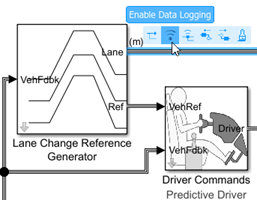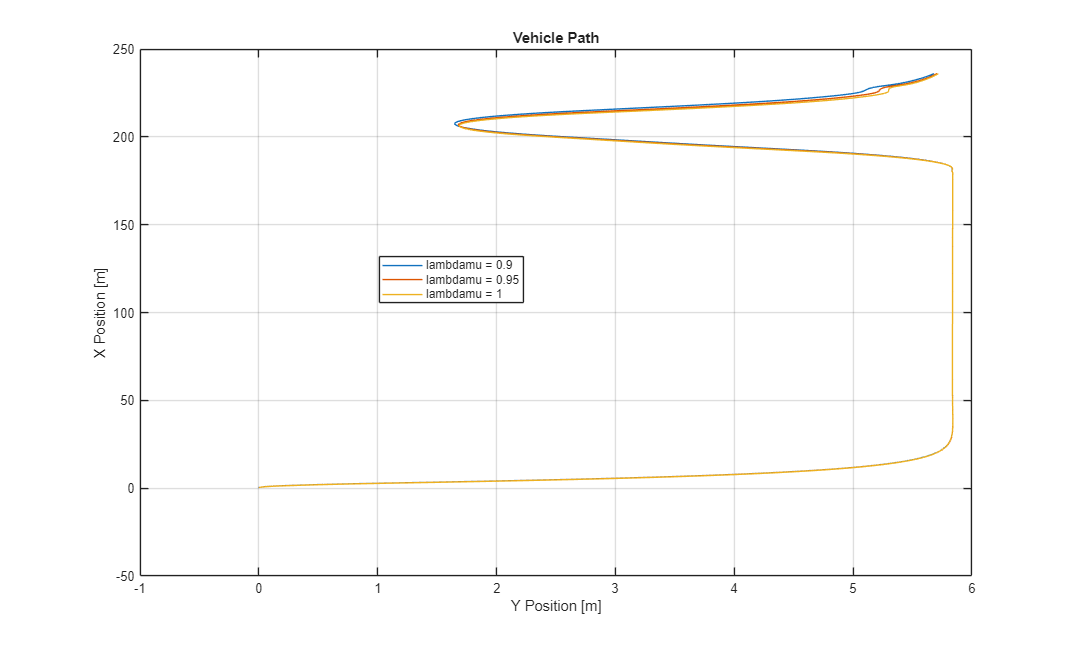# Yaw Stability on Varying Road Surfaces

This example shows how to run the vehicle dynamics double-lane change maneuver on different road surfaces, analyze the vehicle yaw stability, and determine the maneuver success.

ISO 3888-2 defines the double-lane change maneuver to test the obstacle avoidance performance of a vehicle. In the test, the driver:

• Accelerates until vehicle hits a target velocity

• Releases the accelerator pedal

• Turns steering wheel to follow path into the left lane

• Turns steering wheel to follow path back into the right lane

Typically, cones mark the lane boundaries. If the vehicle and driver can negotiate the maneuver without hitting a cone, the vehicle passes the test.

```helpersetupdlc; ```### Run a Double-Lane Change Maneuver

1. Open the Lane Change Reference Generator block. By default, the maneuver is set with these parameters:

• Longitudinal entrance velocity setpoint — 35 mph

• Vehicle width — 2 m

• Lateral reference position breakpoints and Lateral reference data — Values that specify the lateral reference trajectory as a function of the longitudinal distance

2. In the Visualization subsystem, open the 3D Engine block. By default, the 3D Engine parameter is set to Disabled. For the 3D visualization engine platform requirements and hardware recommendations, see the 3D Visualization Engine Requirements and Limitations.

3. Run the maneuver. As the simulation runs, view the vehicle information.

```mdl = 'DLCReferenceApplication'; sim(mdl); ```
```### Starting serial model reference simulation build Warning: method not found. ### Successfully updated the model reference simulation target for: Driveline ### Successfully updated the model reference simulation target for: PassVeh14DOF ### Successfully updated the model reference simulation target for: SiMappedEngineV Build Summary Simulation targets built: Model Action Rebuild Reason ========================================================================================== Driveline Code generated and compiled Driveline_msf.mexw64 does not exist. PassVeh14DOF Code generated and compiled PassVeh14DOF_msf.mexw64 does not exist. SiMappedEngineV Code generated and compiled SiMappedEngineV_msf.mexw64 does not exist. 3 of 3 models built (0 models already up to date) Build duration: 0h 4m 10.411s ```• In the Vehicle Position window, view the vehicle longitudinal distance as a function or the lateral distance.

• In the Visualization subsystem, open the Lane Change scope block to display the lateral displacement as a function of time. The red and orange lines mark the cone boundaries. The blue line marks the reference trajectory and the green line marks the actual trajectory. The green line does come close to the red line that marks the cones.• In the Visualization subsystem, if you enable the 3D Engine block visualization environment, you can view the vehicle response in the `AutoVrtlEnv` window.### Sweep Surface Friction

Run the reference application on three road surfaces with different friction scaling coefficients. Use the results to analyze the yaw stability and help determine the success of the maneuver.

1. In the double-lane change reference application model DLCReferenceApplication, open the Environment subsystem. The Friction block parameter Constant value specifies the friction scaling coefficient. By default, the friction scaling coefficient is `1.0`. The reference application uses the coefficient to adjust the friction at every time step.

2. Enable signal logging for the velocity, lane, and ISO signals. You can use the Simulink® editor or, alternatively, these MATLAB® commands. Save the model.

• Enable signal logging for the Lane Change Reference Generator outport Lane signal.```mdl = 'DLCReferenceApplication'; ph=get_param('DLCReferenceApplication/Lane Change Reference Generator','PortHandles'); set_param(ph.Outport(1),'DataLogging','on'); ```
• Enable signal logging for the Passenger Vehicle block outport signal.```ph=get_param('DLCReferenceApplication/Passenger Vehicle','PortHandles'); set_param(ph.Outport(1),'DataLogging','on'); ```
• In the Visualization subsystem, enable signal logging for the ISO block.```set_param([mdl '/Visualization/ISO 15037-1:2006'],'Measurement','Enable'); ```

3. Set up a vector with the friction scaling coefficients, `lambdamu`, that you want to investigate. For example, to examine friction scaling coefficients equal to `0.9`, `0.95`, and `1.0`, at the command line enter:

```lambdamu = [0.9, 0.95, 1.0]; numExperiments = length(lambdamu); ```

4. Create an array of simulation inputs that sets `lambdamu` equal to the Friction constant block parameter.

```for idx = numExperiments:-1:1 in(idx) = Simulink.SimulationInput(mdl); in(idx) = in(idx).setBlockParameter([mdl '/Environment/Friction'],... 'Value',['ones(4,1).*',num2str(lambdamu(idx))]); end ```

5. Set the simulation stop time at 25 s. Save the model and run the simulations. If available, use parallel computing.

```set_param(mdl,'StopTime','25') save_system(mdl) tic; simout = parsim(in,'ShowSimulationManager','on'); toc; ```
```[17-Dec-2020 13:57:24] Checking for availability of parallel pool... [17-Dec-2020 13:57:24] Starting Simulink on parallel workers... [17-Dec-2020 13:57:25] Loading project on parallel workers... [17-Dec-2020 13:57:25] Configuring simulation cache folder on parallel workers... [17-Dec-2020 13:57:25] Loading model on parallel workers... [17-Dec-2020 13:57:45] Running simulations... [17-Dec-2020 13:58:19] Completed 1 of 3 simulation runs [17-Dec-2020 13:58:20] Completed 2 of 3 simulation runs [17-Dec-2020 13:59:11] Completed 3 of 3 simulation runs [17-Dec-2020 13:59:11] Cleaning up parallel workers... Elapsed time is 120.449498 seconds. ```6. After the simulations complete, close the Simulation Data Inspector windows.

### Use Simulation Data Inspector to Analyze Results

Use the Simulation Data Inspector to examine the results. You can use the UI or, alternatively, command-line functions.

1. Open the Simulation Data Inspector. On the Simulink Toolstrip, on the Simulation tab, under Review Results, click Data Inspector.

• In the Simulation Data Inspector, select Import.• In the Import dialog box, clear `logsout`. Select `simout(1)`, `simout(2)`, and `simout(3)`. Select Import.• Use the Simulation Data Inspector to examine the results.

2. Alternatively, use these MATLAB commands to create 6 plots. The first three plots mark the upper lane boundary, `UB`, lower lane boundary, `LB`, and lateral vehicle distance, `Y`, for each run.

The next three plots provide the lateral acceleration, `ay`, lateral vehicle distance, `Y`, and yaw rate, `r`, for each run.

```for idx = 1:numExperiments % Create sdi run object simoutRun(idx)=Simulink.sdi.Run.create; simoutRun(idx).Name=['lambdamu = ', num2str(lambdamu(idx))]; add(simoutRun(idx),'vars',simout(idx)); end sigcolor=[1 0 0]; for idx = 1:numExperiments % Extract the maneuver upper and lower lane boundaries ubsignal(idx)=getSignalByIndex(simoutRun(idx),1); ubsignal(idx).LineColor = sigcolor; lbsignal(idx)=getSignalByIndex(simoutRun(idx),2); lbsignal(idx).LineColor = sigcolor; end sigcolor=[0 1 0;0 0 1;1 0 1]; for idx = 1:numExperiments % Extract the lateral acceleration, position, and yaw rate ysignal(idx)=getSignalByIndex(simoutRun(idx),27); ysignal(idx).LineColor =sigcolor((idx),:); rsignal(idx)=getSignalByIndex(simoutRun(idx),77); rsignal(idx).LineColor =sigcolor((idx),:); asignal(idx)=getSignalByIndex(simoutRun(idx),79); asignal(idx).LineColor =sigcolor((idx),:); end Simulink.sdi.view Simulink.sdi.setSubPlotLayout(numExperiments,2); for idx = 1:numExperiments % Plot the lateral position and lane boundaries plotOnSubPlot(ubsignal(idx),(idx),1,true); plotOnSubPlot(lbsignal(idx),(idx),1,true); plotOnSubPlot(ysignal(idx),(idx),1,true); end for idx = 1:numExperiments % Plot the lateral acceleration, position, and yaw rate plotOnSubPlot(asignal(idx),1,2,true); plotOnSubPlot(ysignal(idx),2,2,true); plotOnSubPlot(rsignal(idx),3,2,true); end ```

The results are similar to these plots, which indicate that the vehicle has a yaw rate of about `.66` rad/s when the friction scaling coefficient is equal to `1`.### Further Analysis

To explore the results further, use these commands to extract the lateral acceleration, steering angle, and vehicle trajectory from the `simout` object.

1. Extract the lateral acceleration and steering angle. Plot the data. The results are similar to this plot. They indicate that the greatest lateral acceleration occurs when the friction scaling coefficient is `1`.

```figure for idx = 1:numExperiments % Extract Data log = get(simout(idx),'logsout'); sa=log.get('Steering-wheel angle').Values; ay=log.get('Lateral acceleration').Values; legend_labels{idx} = ['lambdamu = ', num2str(lambdamu(idx))]; % Plot steering angle vs. lateral acceleration plot(sa.Data,ay.Data) hold on end % Add labels to the plots legend(legend_labels, 'Location', 'best'); title('Lateral Acceleration') xlabel('Steering Angle [deg]') ylabel('Acceleration [m/s^2]') grid on ```2. Extract the vehicle path. Plot the data. The results are similar to this plot. They indicate that the greatest lateral vehicle position occurs when the friction scaling coefficient is `0.9`.

```figure for idx = 1:numExperiments % Extract Data log = get(simout(idx),'logsout'); x = log{3}.Values.Body.InertFrm.Cg.Disp.X.Data; y = log{3}.Values.Body.InertFrm.Cg.Disp.Y.Data; legend_labels{idx} = ['lambdamu = ', num2str(lambdamu(idx))]; % Plot vehicle location plot(y,x) hold on end % Add labels to the plots legend(legend_labels, 'Location', 'best'); title('Vehicle Path') xlabel('Y Position [m]') ylabel('X Position [m]') grid on ```